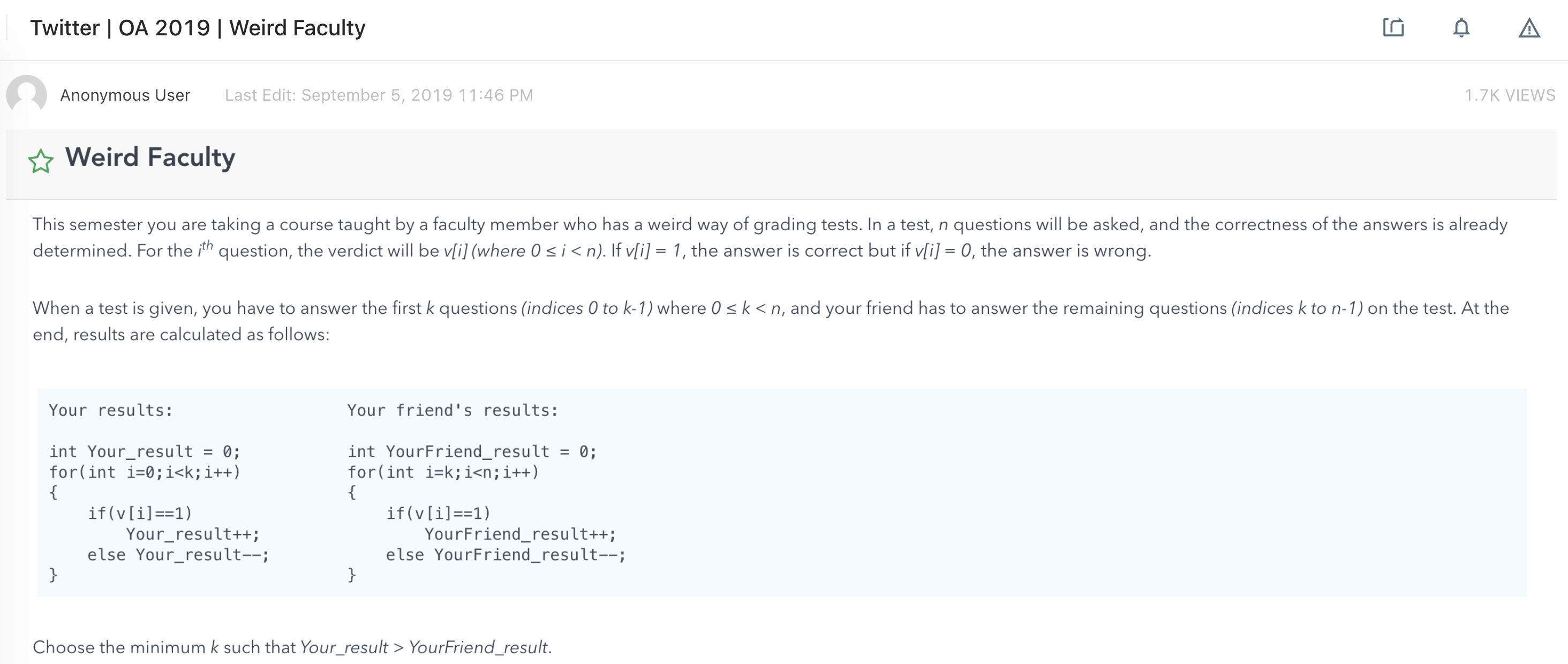Reference: LeetCode
Difficulty: Medium

## Problem

Given a list of daily temperatures T, return a list such that, for each day in the input, tells you how many days you would have to wait until a warmer temperature. If there is no future day for which this is possible, put 0 instead.

Note: The length of temperatures will be in the range [1, 30000]. Each temperature will be an integer in the range [30, 100].

Example:

## Analysis

### Brute-Force

For each temperature, we find its first strictly increasing temperature.

Time: $O(N^2)$
Space: $O(1)$

### Stack

We use a stack to keep track of a list of strictly increasing temperatures.

From LeetCode solution:Note: Check stack’s size before popping.

Time: $O(N)$ since each index gets pushed ad popped at most once from the stack. good!
Space: $O(W)$ where $W$ is the size of the stack bounded by the number of temperatures that are strictly increasing.

CommentJunhao Wang
Hi, I was a master student at USC studying Computer Science.